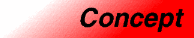SortedAssociativeContainerCategory: containers Component type: concept

Description

A Sorted Associative Container is a type of AssociativeContainer. Sorted Associative Containers use an ordering relation on their keys; two keys are considered to be equivalent if neither one is less than the other. (If the ordering relation is case-insensitive string comparison, for example, then the keys "abcde" and "aBcDe" are equivalent.)

Sorted Associative Containers guarantee that the complexity for most operations is never worse than logarithmic , and they also guarantee that their elements are always sorted in ascending order by key.

Associated types

Two new types are introduced, in addition to the types defined in the AssociativeContainer and ReversibleContainer requirements.

 X::key_compare The type of a StrictWeakOrdering used to compare keys. Its argument type must be X::key_type. X::value_compare The type of a StrictWeakOrdering used to compare values. Its argument type must be X::value_type, and it compares two objects of value_type by passing the keys associated with those objects to a function object of type key_compare.

Notation

 X A type that is a model of Sorted Associative Container a Object of type X t Object of type X::value_type k Object of type X::key_type p, q Object of type X::iterator c Object of type X::key_compare

Valid expressions

In addition to the expressions defined in AssociativeContainer and ReversibleContainer, the following expressions must be valid.

Name Expression Type requirements Return type
Default constructor
X()
X a;

Constructor with compare
X(c)
X a(c);

Key comparison a.key_comp()   X::key_compare
Value comparison a::value_compare()   X::value_compare
Lower bound a.lower_bound(k)   iterator if a is mutable, otherwise const_iterator.
Upper bound a.upper_bound(k)   iterator if a is mutable, otherwise const_iterator.
Equal range a.equal_range(k)   pair<iterator, iterator> if a is mutable, otherwise pair<const_iterator, const_iterator>.

Expression semantics

Name Expression Precondition Semantics Postcondition
Default constructor
X()
X a;
Creates an empty container, using key_compare() as the comparison object. The size of the container is 0.
Constructor with compare
X(c)
X a(c);
Creates an empty container, using c as the comparison object. The size of the container is 0. key_comp() returns a function object that is equivalent to c.
Key comparison a.key_comp()   Returns the key comparison object used by a.
Value comparison a::value_compare()   Returns the value comparison object used by a. If t1 and t2 are objects of type value_type, and k1 and k2 are the keys associated with them, then a.value_comp()(t1, t2) is equivalent to a.key_comp()(k1, k2).
Lower bound a.lower_bound(k)   Returns an iterator pointing to the first element whose key is not less than k. Returns a.end() if no such element exists. If a contains any elements that have the same key as k, then the return value of lower_bound points to the first such element.
Upper bound a.upper_bound(k)   Returns an iterator pointing to the first element whose key is greater than k. Returns a.end() if no such element exists. If a contains any elements that have the same key as k, then the return value of upper_bound points to one past the last such element.
Equal range a.equal_range(k)   Returns a pair whose first element is a.lower_bound(k) and whose second element is a.upper_bound(k).

Complexity guarantees

key_comp() and value_comp() are constant time.

Erase element is constant time.

Erase key is O(log(size()) + count(k)). 

Erase range is O(log(size()) + N), where N is the length of the range. 

Find is logarithmic. 

Count is O(log(size()) + count(k)). 

Lower bound, upper bound, and equal range are logarithmic. 

Invariants

 Definition of value_comp If t1 and t2 are objects of type X::value_type and k1 and k2 are the keys associated with those objects, then a.value_comp() returns a functors such that a.value_comp()(t1, t2) is equivalent to a.key_comp()(k1, k2). Ascending order The elements in a Sorted Associative Container are always arranged in ascending order by key. That is, if a is a Sorted Associative Container, then is_sorted(a.begin(), a.end(), a.value_comp()) is always true.